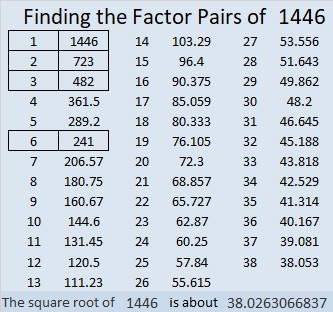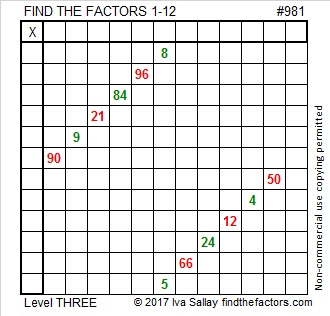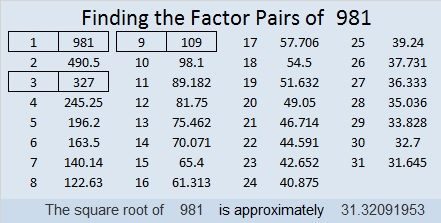# 1446 Peppermint Stick

Red and green striped peppermint sticks are often seen in stores and homes in December. Can you lick this peppermint stick puzzle or will you let it lick you?The puzzle number was 1446. Here are a few facts about that number:

• 1446 is a composite number.
• Prime factorization: 1446 = 2 × 3 × 241
• 1446 has no exponents greater than 1 in its prime factorization, so √1446 cannot be simplified.
• The exponents in the prime factorization are 1, 1, and 1. Adding one to each exponent and multiplying we get (1 + 1)(1 + 1)(1 + 1) = 2 × 2 × 2 = 8. Therefore 1446 has exactly 8 factors.
• The factors of 1446 are outlined with their factor pair partners in the graphic below.1446 is also the hypotenuse of a Pythagorean triple:
720-1254-1446 which is 6 times (120-209-241)

# 981 Peppermint Sticks

This time of year you can buy peppermint sticks that don’t just have red stripes, but they might have green ones, too. Today’s puzzle looks like a couple of peppermint sticks. It will be a sweet experience for you to solve it, so be sure to give it a try.Print the puzzles or type the solution in this excel file: 12 factors 978-985

Here is some information about the number 981:

30² + 9² = 981 making 981 the hypotenuse of a Pythagorean triple:
540-819-981 which is 9 times (60-91-109) but can also be calculated from
2(30)(9), 30² – 9², 30² + 9²

981 is palindrome 171 in BASE 28 because 1(28²) + 7(28) + 1(1) = 981

• 981 is a composite number.
• Prime factorization: 981 = 3 × 3 × 109, which can be written 981 = 3² × 109
• The exponents in the prime factorization are 2 and 1. Adding one to each and multiplying we get (2 + 1)(1 + 1) = 3 × 2  = 6. Therefore 981 has exactly 6 factors.
• Factors of 981: 1, 3, 9, 109, 327, 981
• Factor pairs: 981 = 1 × 981, 3 × 327, or 9 × 109
• Taking the factor pair with the largest square number factor, we get √981 = (√9)(√109) = 3√109 ≈ 31.3209